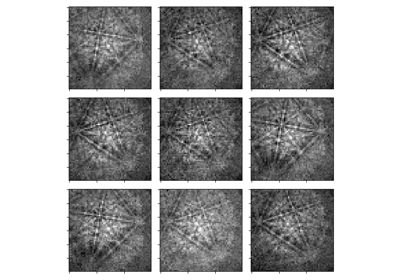# crop#

EBSD.crop(*args, **kwargs)[source]#

Crops the data in a given axis. The range is given in pixels.

This docstring was copied from HyperSpy’s Signal2D.crop. Some inconsistencies with the kikuchipy version may exist.

Parameters:
axis

Specify the data axis in which to perform the cropping operation. The axis can be specified using the index of the axis in axes_manager or the axis name.

start`int`, `float`, `or` `None`

The beginning of the cropping interval. If type is `int`, the value is taken as the axis index. If type is `float` the index is calculated using the axis calibration. If start/end is `None` the method crops from/to the low/high end of the axis.

end`int`, `float`, `or` `None`

The end of the cropping interval. If type is `int`, the value is taken as the axis index. If type is `float` the index is calculated using the axis calibration. If start/end is `None` the method crops from/to the low/high end of the axis.

convert_unitsbool

Default is `False`. If `True`, convert the units using the `convert_units()` method of the `AxesManager`. If `False`, does nothing.

## Examples using `EBSD.crop`#